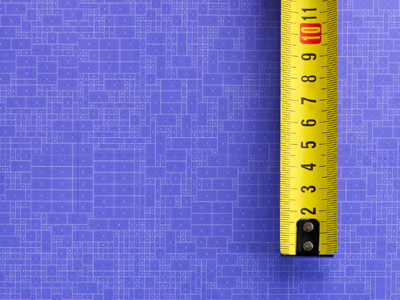The metric system is based on decimal numbers: 10 mm = 1 cm, 100 cm = 1 m, 1,000 m = 1 km.

# Decimal Numbers 3 (Medium)

Welcome to the third quiz in our Medium section of Eleven Plus maths quizzes on Decimal Numbers. If you have played the previous ones, then you should sail through this, armed with what you have already learned. We’ll ask you to convert decimals into fractions, and vice versa, and we’ll also see whether you know the rules when multiplying or dividing decimals by multiples of ten.

Here’s a recap:

• When dividing, move the digits to the right
• When multiplying, move the digits to the left
• If you divide/multiply by 10, move the digits one place to the left/right
• If you divide/multiply by 100, move the digits two places to the left/right
• If you divide/multiply by 1,000, move the digits three places to the left/right

Feeling confident? Then on with the quiz. Have fun!

1.
If you divide 78.604 by 100, what number do you get?
7,860.4
7.8604
786.04
0.78604
If you divide by 100, move the digits two places to the right
2.
What is ‘seven-eighths' as a decimal?
0.875
0.775
0.979
0.85
78 = 0.875: divide 7 by eight
3.
If you divide 101.742 by 1,000, what number do you get?
1,017.42
10.1742
1.01742
0.101742
If you divide by 1,000, move the digits 3 places to the right. The decimal point in whole numbers cannot be seen, but it can be imagined as coming straight after the number, followed by zeros (i.e. 221 = 221.000)
4.
If you multiply 753.002 by 10, what number do you get?
75.3002
7,530.02
7.53002
75,300.2
If you multiply by 10, move the digits one place to the left
5.
What is 1.2 as a fraction?
114
115
116
117
0.2 = 15, so 1.2 will equal 115
6.
If you turn ‘three-quarters’ into a decimal and then multiply by 100, what is the answer?
0.75
7.5
75
750
3 ÷ 4 = 0.75 and 0.75 x 100 = 75
7.
If you multiply 0.986 by 100, what number do you get?
9.86
0.0986
98.6
986
If you multiply by 100, move the digits two places to the left
8.
What is ‘seven-thirds' as a decimal?
0.429
2.333
1.666
2.666
73 = 2.333: divide seven by three
9.
If you multiply 0.109 by 1,000, what number do you get?
1,090
1.09
10.9
109
If you multiply by 1,000, move the digits 3 places to the left
10.
If you divide 0.023 by 10, what number do you get?
0.0023
2.3
0.23
23
If you divide by 10, move the digits one place to the right
Author:  Frank Evans

We use cookies to make your experience of our website better.

To comply with the new e-Privacy directive, we need to ask for your consent -